C++ Program to Find Size of int, float, double and char in Your System

In this tutorial you will learn about the C++ Program to Find Size of int, float, double and char in Your System and its application with practical example.

In this tutorial, we will learn to create a C++ program that will Find Size of int, float, double and char in Your System using C++ programming.

Prerequisites

Before starting with this tutorial we assume that you are best aware of the following C++ programming topics:

• Operators in C++ Programming.
• Basic Input and Output function in C++ Programming.
• Basic C++ programming.
• Arithmetic operations in C++ Programming.

What is a data type?

A data type, in every programming language, specifies which type of value a variable is going to hold in the program. i.e. integer, character, float, double.

Program to check the size of predefined data types in C++ programming.

In every programming language, there are many predefined datatypes available. Today we will create a program that will print the size of integer, character, float, and double in bytes. Because every datatype variable reserves the size in memory for each type of variable.

With the help of this program, we can find the size of the above data types.

Output:-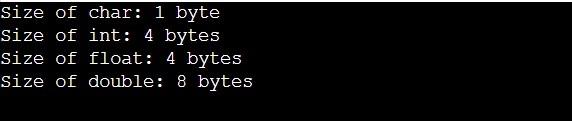Printing the size of character data type using the size of function.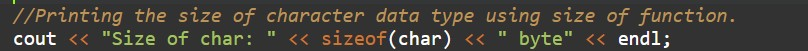Integer type printing its size by using sizeof function.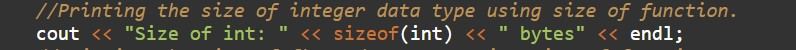Printing the size of float data type using the size of function.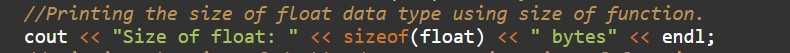Printing the size of double data type using the size of function.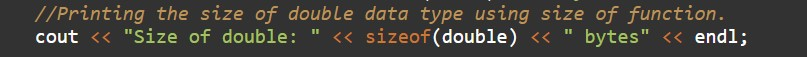In this tutorial we have learn about the C++ Program to Find Size of int, float, double and char in Your System and its application with practical example. I hope you will like this tutorial.